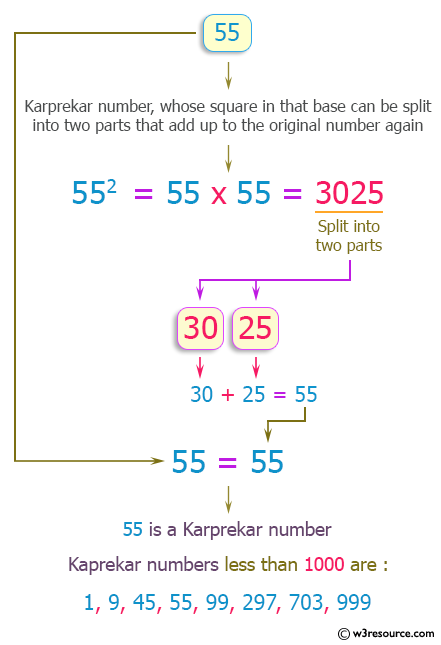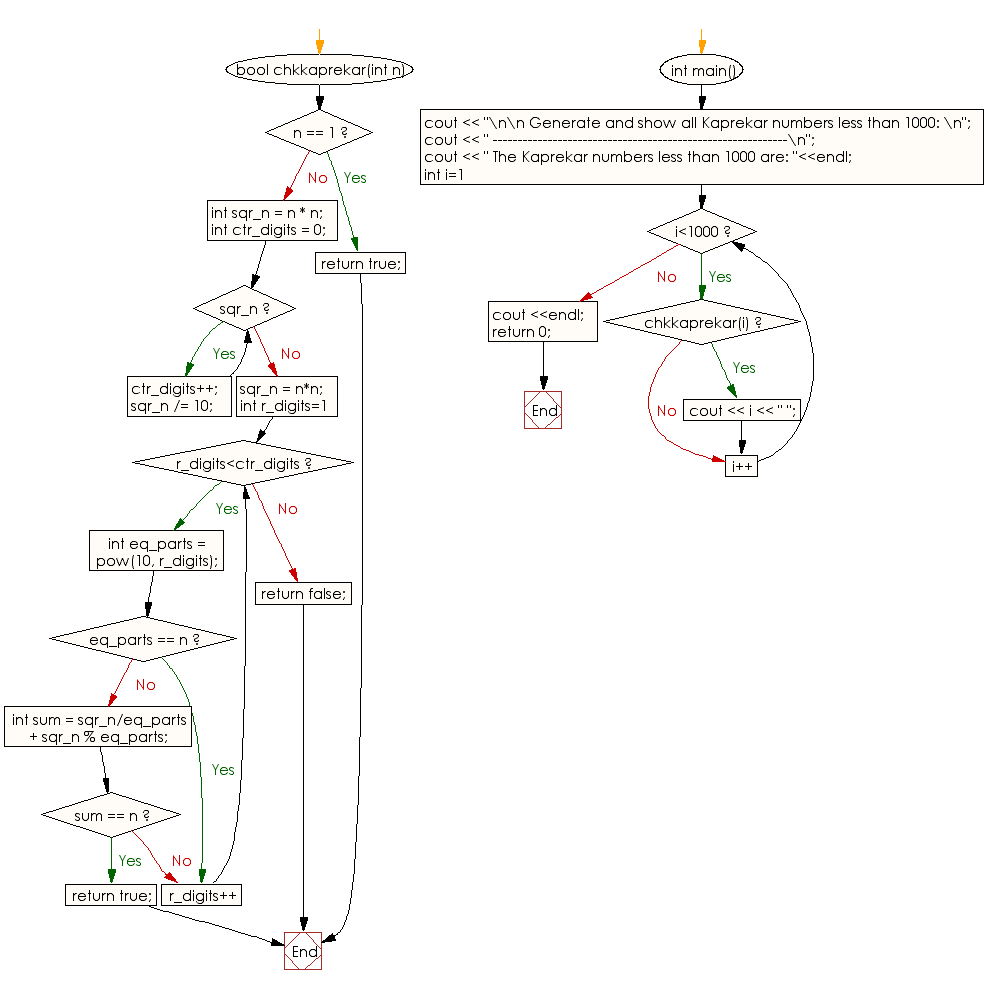﻿ C++ : Generate and show all Kaprekar numbers less than 1000

# C++ Exercises: Generate and show all Kaprekar numbers less than 1000

## C++ Numbers: Exercise-10 with Solution

Write a program in C++ to generate and show all Kaprekar numbers less than 1000.

Pictorial Presentation:Sample Solution:

C++ Code :

``````#include<bits/stdc++.h>
using namespace std;

bool chkkaprekar(int n)
{
if (n == 1)
return true;

int sqr_n = n * n;
int ctr_digits = 0;
while (sqr_n)
{
ctr_digits++;
sqr_n /= 10;
}

sqr_n = n*n;

for (int r_digits=1; r_digits<ctr_digits; r_digits++)
{
int eq_parts = pow(10, r_digits);

if (eq_parts == n)
continue;

int sum = sqr_n/eq_parts + sqr_n % eq_parts;
if (sum == n)
return true;
}
return false;
}
int main()
{
cout << "\n\n Generate and show all Kaprekar numbers less than 1000: \n";
cout << " -----------------------------------------------------------\n";
cout << " The Kaprekar numbers less than 1000 are: "<<endl;
for (int i=1; i<1000; i++)
{
if (chkkaprekar(i))
{
cout << i << " ";

}

}
cout <<endl;
return 0;
}
``````

Sample Output:

``` Generate and show all Kaprekar numbers less than 1000:
-----------------------------------------------------------
The Kaprekar numbers less than 1000 are:
1 9 45 55 99 297 703 999
```

Flowchart:C++ Code Editor:

What is the difficulty level of this exercise?

﻿

## C++ Programming: Tips of the Day

How to use doxygen to create UML class diagrams from C++ source

Doxygen creates inheritance diagrams but I dont think it will create an entire class hierachy. It does allow you to use the GraphViz tool. If you use the Doxygen GUI frontend tool you will find the relevant options in Step2: -> Wizard tab -> Diagrams. The DOT relation options are under the Expert Tab.

Ref: https://bit.ly/3QUFLBY

We are closing our Disqus commenting system for some maintenanace issues. You may write to us at reach[at]yahoo[dot]com or visit us at Facebook